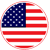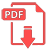# Home | Mathematics | Quadratic equation

## Second-Degree Equation

The complete second degree equations or quadratic equations are represented as follows:

ax2 + bx +c

Where a, b and c are the constants of the equation:

a is the number that always goes in front of x squared
b is the number that always goes in front of the x
c is the number without unknow

That is, the complete second degree equations are those that have an endpoint with x elevated to 2, term with x elevated to 1 (or simply x). If any of these terms are missing, we would be talking about incomplete second-degree equations, which are solved by a different procedure.

As second-degree equations, they have 2 solutions. Remember that the degree of an equation is equal to the number of solutions.English